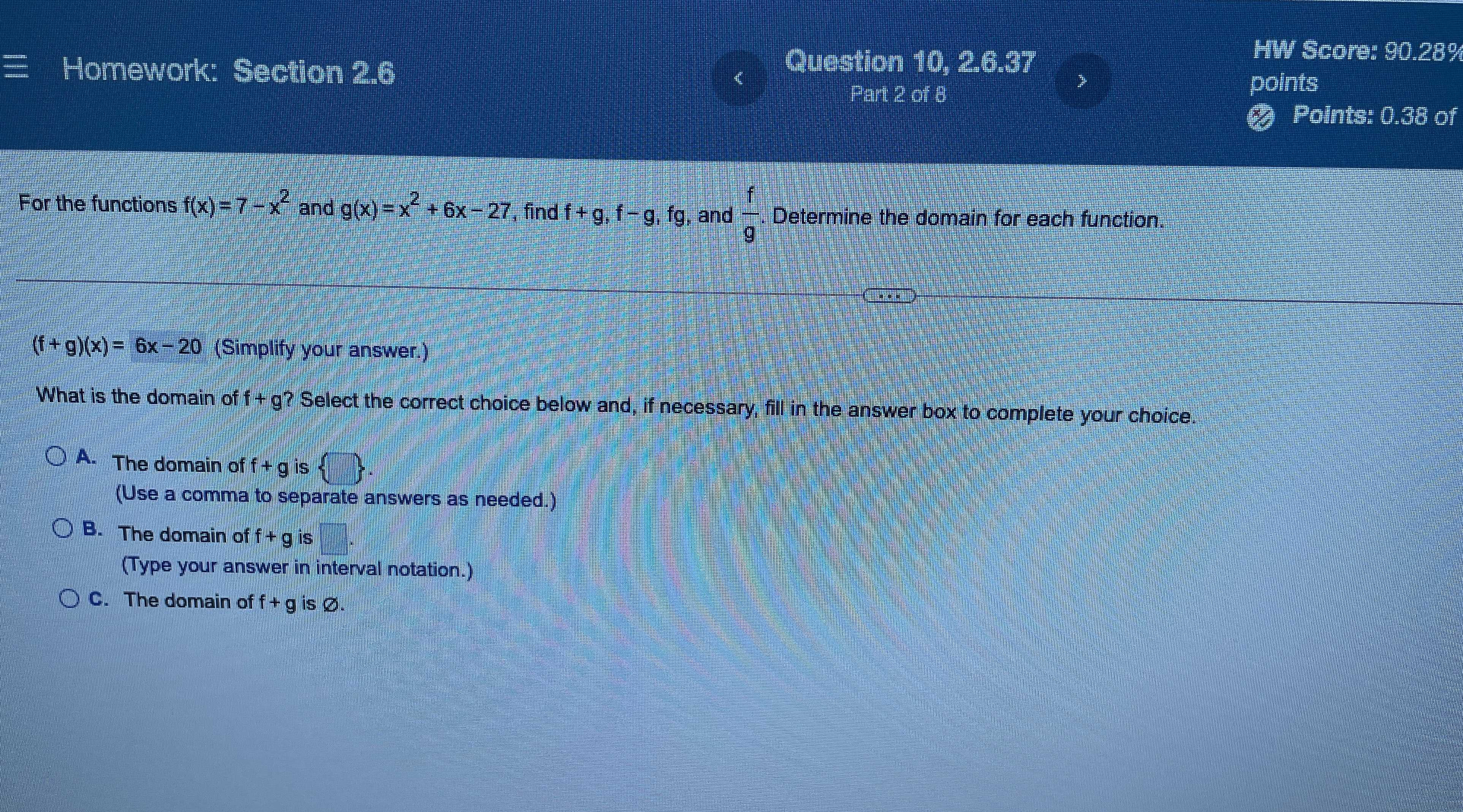### Still have math questions?

Algebra
QuestionFor the functions $$f ( x ) = 7 - x ^ { 2 }$$ and $$g ( x ) = x ^ { 2 } + 6 x - 27 ,$$ find $$f + g , f - g$$ , fg, and $$\frac { f } { g }$$ . Determine the domain for each function.

$$( f + g ) ( x ) = 6 x - 20$$ (Simplify your answer.)

What is the domain of $$f + g ?$$ Select the correct choice below and, if necessary, fill in the answer box to complete your choice.

A. The domain of $$f + g$$ is_____ .

(Use a comma to separate answers as needed.)

B. The domain of $$f + g$$ is______.

C. The domain of $$f + g$$ is ____.
B. $$- \infty < x< \infty$$